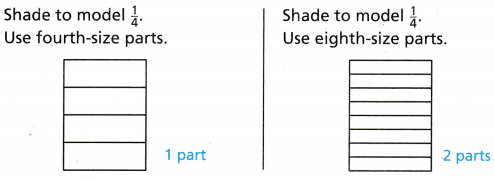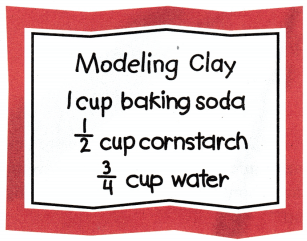# Texas Go Math Grade 4 Lesson 3.2 Answer Key Generate Equivalent Fractions

Refer to our Texas Go Math Grade 4 Answer Key Pdf to score good marks in the exams. Test yourself by practicing the problems from Texas Go Math Grade 4 Lesson 3.2 Answer Key Generate Equivalent Fractions.

## Texas Go Math Grade 4 Lesson 3.2 Answer Key Generate Equivalent Fractions

Essential Question

How con you use multiplication to find equivalent fractions?

Unlock the Problem

Patty needs $$\frac{3}{4}$$ cup of dish soap to make homemade bubble solution. Her measuring cup is divided into eighths. What fraction of the measuring cup should Patty fill with dish soap?

• Is an eighth-size part of a measuring cup bigger or smaller than a fourth-size part?

Find how many eighths are in $$\frac{3}{4}$$

STEP 1 Compare fourths and eighths.You need _________ eighth-size parts to make 1 fourth-size part.

STEP 2. Find how many eighths you need to make 3 fourths.You needed 2 eighth-size parts to make 1 fourth-size part.
So, you need __________ eighth-size parts to make 3 fourth-size parts.
So, Patty should fill $$\frac{}{8}$$ of the measuring cup with dish soap.

Math Talk

Mathematical Processes
How did you know how many eighth-size parts you needed to make 1 fourth-size part? Explain.

Question 1.
Explain why 6 eighth-size parts is the same amount as 3 fourth-size parts.

Example Write two fractions that are equivalent to $$\frac{1}{2}$$So, $$\frac{1}{2}$$ = ________ = ________

Question 2.
Look at the model that shows $$\frac{1}{2}$$ = $$\frac{3}{6}$$ How does the number of parts in the whole affect the number of parts that are shaded? Explain.

Math Talk

Mathematical Processes
Explain how you can use multiplication to write a fraction that is equivalent to $$\frac{3}{5}$$

Share and Show

Question 1.
write a fraction that is equivalent to $$\frac{1}{3}$$.Write two equivalent fractions.

Question 2.Question 3.Problem Solving

Use the recipe for 4-6.Question 4.
Apply How could you use a $$\frac{1}{8}$$-cup measuring cup to measure the cornstarch?

Question 5.
How could you use a $$\frac{1}{8}$$-cup measuring cup to measure the water?

Question 6.
H.O.T. Kim says the amount of flour in the recipe can be expressed as a fraction. Is she correct? Explain.

Question 7.
Write Math Explain using words how you know a fraction is equivalent to another fraction.

Question 8.
Multi-Step Marcus needs $$\frac{3}{4}$$ cup of sugar. Marcus said he could not measure out the sugar using a $$\frac{1}{6}$$-cup measuring cup. Explain why not and suggest what size measuring cup will work.

Question 9.
Ten trees are growing in Cindy’s yard. Of those, $$\frac{3}{5}$$ are pine trees. Which fraction is equivalent to $$\frac{3}{5}$$?
(A) $$\frac{10}{10}$$
(B) $$\frac{5}{10}$$
(C) $$\frac{6}{10}$$
(D) $$\frac{1}{10}$$

Question 10.
Clara read $$\frac{2}{3}$$ of a book. Which fraction is equivalent to what Clara read?
(A) $$\frac{5}{6}$$
(B) $$\frac{4}{9}$$
(C) $$\frac{6}{9}$$
(D) $$\frac{2}{6}$$

Question 11.
Multi-Step Ana uses equivalent amounts of seeds and corn to fill her bird feeder. Which could be the amount of seeds and corn that Ana uses?
(A) $$\frac{1}{4}$$ cup seeds, $$\frac{1}{8}$$ cup corn
(B) $$\frac{1}{4}$$ cup seeds, $$\frac{2}{8}$$ cup corn
(C) $$\frac{3}{4}$$ cup seeds, $$\frac{3}{8}$$ cup corn
(D) $$\frac{3}{4}$$ cup seeds, $$\frac{7}{8}$$ cup corn

TEXAS Test Prep

Question 12.
Raul needs a piece of rope $$\frac{2}{3}$$yard long. Which fraction is equivalent to $$\frac{2}{3}$$?
(A) $$\frac{8}{15}$$
(B) $$\frac{6}{12}$$
(C) $$\frac{8}{12}$$
(D) $$\frac{4}{5}$$

### Texas Go Math Grade 4 Lesson 3.2 Homework and Practice Answer Key

Write two equivalent fractions.

Question 1.Question 2.Problem Solving

Use the recipe for 3-4.Question 3.
How could you use a $$\frac{1}{4}$$-cup measuring cup to measure the water?

Question 4.
How could you use a $$\frac{1}{4}$$-cup measuring cup to measure the cornstarch?

Lesson Check

Question 5.
Which two fractions are equivalent to $$\frac{3}{10}$$?
(A) $$\frac{6}{30}, \frac{9}{10}$$
(B) $$\frac{1}{5}, \frac{3}{15}$$
(C) $$\frac{6}{20}, \frac{9}{30}$$
(D) $$\frac{12}{14}, \frac{6}{7}$$

Question 6.
Sophie needs a piece of rope that is $$\frac{3}{4}$$ yard long. Which fraction is equivalent to $$\frac{3}{4}$$?
(A) $$\frac{9}{10}$$
(B) $$\frac{4}{3}$$
(C) $$\frac{4}{5}$$
(D) $$\frac{6}{12}$$

Question 7.
Theo needs to measure $$\frac{3}{4}$$ cup of water He has a $$\frac{1}{8}$$-cup measuring cup. How many $$\frac{1}{8}$$ cups are in $$\frac{3}{4}$$ cup?
(A) 4
(B) 8
(C) 6
(D) 3

Question 8.
Sandra finished $$\frac{1}{3}$$ of her homework problems. Which fraction is equivalent to what Sandra finished?
(A) $$\frac{4}{6}$$
(B) $$\frac{2}{6}$$
(C) $$\frac{3}{8}$$
(D) $$\frac{1}{6}$$

Question 9.
Multi-Step Paul needs to measure $$\frac{1}{4}$$ cup lemon juice to make lemonade. how many times should he fill his $$\frac{1}{8}$$-cup measuring cup?
(A) four times
(B) three times
(C) two times
(D) eight times
Multi-Step Carrie needs $$\frac{1}{3}$$ cup of peanuts and $$\frac{1}{3}$$ cup of walnuts for her trail mix. Which fraction is equivalent to the total amount of nuts Carrie needs for her mix?
(A) $$\frac{2}{6}$$
(B) $$\frac{3}{9}$$
(C) $$\frac{3}{6}$$
(D) $$\frac{6}{9}$$